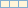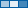# Curriculum and Labs for Engineering Education

cancel
Showing results for
Did you mean:

## Unit 2 - Fundamentals: Lesson 4

Introduction

In this lesson we are going to learn about arrays. We will look at teo methods of creating arrays and different array functions that will make manipulation easy. Finally we will look at the auto-indexing feature available in LabVIEW and how it can help us with arrays.

Lesson Video 1

Example Snippet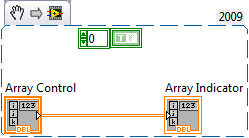Lesson Video 2

Example Snippet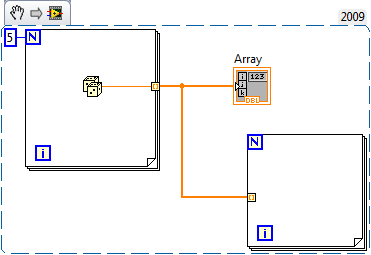Practice Problem

Procedure: Create the required VI(s) to achieve the following tasks.

Requirements:

1. Generate an array of 10 random numbers.
2. Find the maximum, minimum, and mean of the array using a shift register.
3. Modify the array by multiplying the fifth element by 2

Practice Solution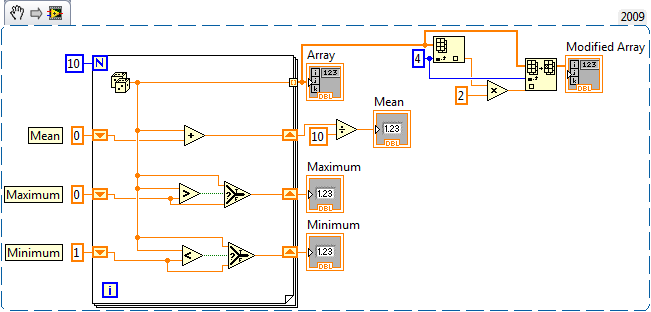Next Step

Unit 3 - Case Structures: Lesson 1